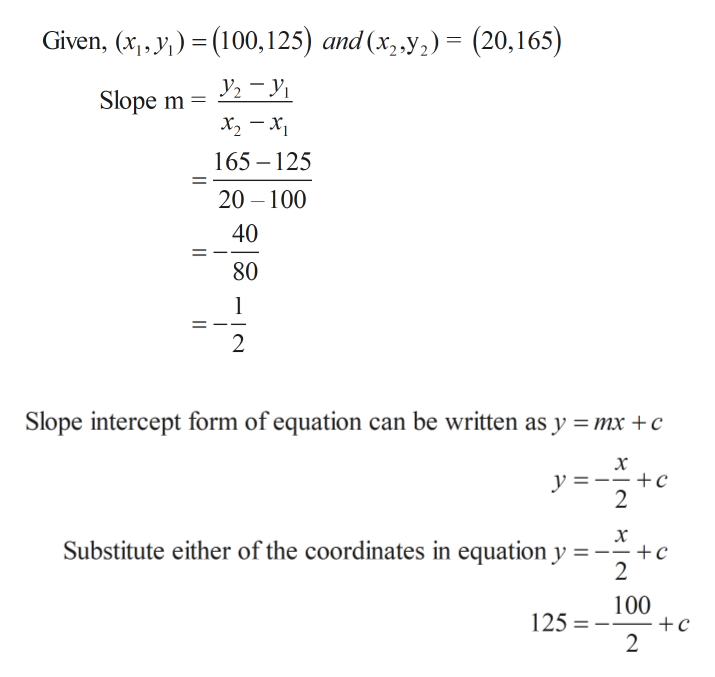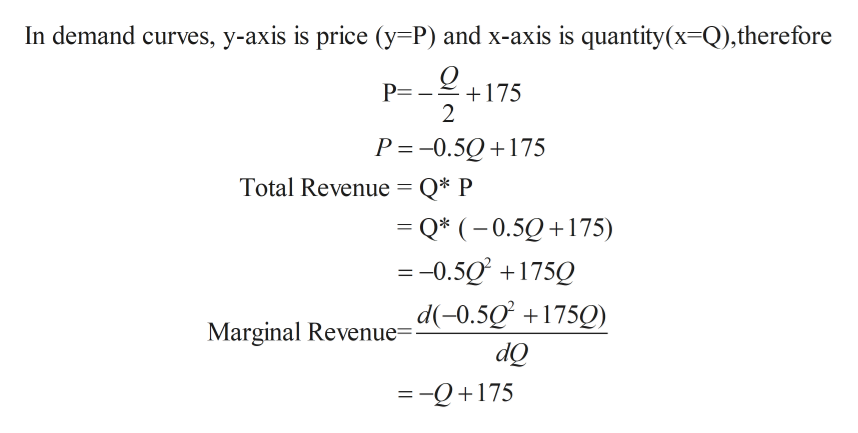# a monopolist finds the demand curve to be linear, containing data points (q,p) of (100,125) and (20,165).a. how many items can he expect to sell, if the price p is \$100?b. what price should he charge to maximize the revenue?

Question
45 views

a monopolist finds the demand curve to be linear, containing data points (q,p) of (100,125) and (20,165).

a. how many items can he expect to sell, if the price p is \$100?

b. what price should he charge to maximize the revenue?

check_circle

Step 1

We know that the Demand Q is linear and passes through data points (100,125) and (20,165).

Demand equation can be calculated using slope-intercept form as shown below.help_outlineImage TranscriptioncloseGiven, (x,, y(100,125) and (x,,y) (20,165) Slope m х, — х, 165 125 20 100 40 80 1 2 Slope intercept form of equation can be written as y mx +c х y = +c 2 х Substitute either of the coordinates in equation y --+c 2 100 125 2 fullscreen
Step 2

Total revenue and Marginal revenue can be calculated as shown below.help_outlineImage TranscriptioncloseIn demand curves, y-axis is price (y=P) and x-axis is quantity(x-Q),therefore P= 175 2 P =-0.5Q175 Total Revenue = Q* P = Q* (0.50175) =-0.5 175Q + Marginal Revenue-d(-0.5Q +175O dQ = -Q+175 fullscreen
Step 3

In monopoly, P is always greater than MR ie P>MR

...

### Want to see the full answer?

See Solution

#### Want to see this answer and more?

Solutions are written by subject experts who are available 24/7. Questions are typically answered within 1 hour.*

See Solution
*Response times may vary by subject and question.
Tagged in